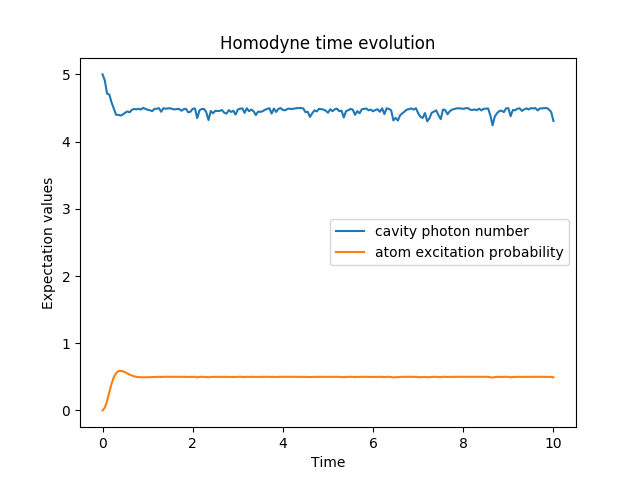# Stochastic Solver¶

## Homodyne detection¶

Homodyne detection is an extension of the photocurrent method where the output is mixed with a strong external source allowing to get information about the phase of the system. With this method, the resulting detection rate depends is

\begin{align}\begin{aligned}:label: jump_rate\\\tau = tr \left((\gamma^2 + \gamma (C+C^\dag) + C^\dag C)\rho \right)\end{aligned}\end{align}

With $$\gamma$$, the strength of the external beam and $$C$$ the collapse operator. When the beam is very strong $$(\gamma >> C^\dag C)$$, the rate becomes a constant term plus a term proportional to the quadrature of the system.

### Closed system¶

In closed systems, the resulting stochastic differential equation is

(1)$\delta \psi(t) = - i H \psi(t) \delta t - \sum_n \left( \frac{C_n^{+} C_n}{2} -\frac{e_n}{2} C_n + \frac{e_n^2}{8} \right) \psi \delta t + \sum_n \left( C_n - \frac{e_n}{2} \right) \psi \delta \omega$

with

\begin{align}\begin{aligned}:label: jump_rate\\e_n = \left<\psi(t)|C_n + C_n^{+}|\psi(t)\right>\end{aligned}\end{align}

Here $$\delta \omega$$ is a Wiener increment.

In QuTiP, this is available with the function ssesolve.

In : times = np.linspace(0.0, 10.0, 201)

In : psi0 = tensor(fock(2, 0), fock(10, 5))

In : a  = tensor(qeye(2), destroy(10))

In : sm = tensor(destroy(2), qeye(10))

In : H = 2 * np.pi * a.dag() * a + 2 * np.pi * sm.dag() * sm + 2 * np.pi * 0.25 * (sm * a.dag() + sm.dag() * a)

In : data = ssesolve(H, psi0, times, sc_ops=[np.sqrt(0.1) * a], e_ops=[a.dag() * a, sm.dag() * sm], method="homodyne")
Total run time:   0.03s

In : figure()
Out: <Figure size 640x480 with 0 Axes>

In : plot(times, data.expect, times, data.expect)
Out:
[<matplotlib.lines.Line2D at 0x2b22694b2cf8>,
<matplotlib.lines.Line2D at 0x2b22694b26a0>]

In : title('Homodyne time evolution')
Out: Text(0.5,1,'Homodyne time evolution')

In : xlabel('Time')
Out: Text(0.5,0,'Time')

In : ylabel('Expectation values')
Out: Text(0,0.5,'Expectation values')

In : legend(("cavity photon number", "atom excitation probability"))
Out: <matplotlib.legend.Legend at 0x2b226a6bd390>

In : show()### Open system¶

In open systems, 2 types of collapse operators are considered, $$S_i$$ represent the dissipation in the environment, $$C_i$$ are monitored operators. The deterministic part of the evolution is the liouvillian with both types of collapses

(2)$L(\rho(t)) = - i[H(t),\rho(t)] + \sum_n D(S_n, \rho) + \sum_i D(C_i, \rho),$

with

(3)$D(C, \rho) = \frac{1}{2} \left[2 C \rho(t) C^{+} - \rho(t) C^{+} C - C^{+} C \rho(t) \right].$

The stochastic part is given by

(4)$d_2 = \left(C \rho(t) + \rho(t) C^{+} - \rm{tr}\left(C \times \rho + \rho \times C^{+} \right)\rho(t) \right),$

resulting in the stochastic differential equation

(5)$\delta \rho(t) = L(\rho(t)) \delta t + d_2 \delta \omega$

The function smesolve covert these cases in QuTiP.

### Heterodyne detection¶

With heterodyne detection, two measurements are made in order to obtain information about 2 orthogonal quadratures at once.